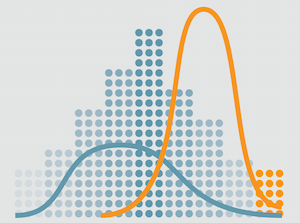# Statistics

These notes closely follow Introduction to Probability and Statistics for Engineers and Scientists by Sheldon Ross; All of Statistics by Larry Wasserman and Statistical Regression from Khan Academy.### Special Distributions

In this note we go over some probability distributions that come up very frequently in the study of statistics, namely Poission distribution, Normal distribution, t-distribution and Chi Squared distribution.

### Sampling Statistics

Here we study some common statistics that arise from a sample (sample mean, sample variance, etc.) and also explore their probability distributions.

### Statistical Inference

We explore what "Statistical Inference" actually means. We formally define "Statistical Models", "Regression Functions", etc. and finally go over the difference between Classical Inference and Bayesian Inference.

### Classical Inference I

Most classical inference problems can be identified as being one of three types - point estimation, interval estimation, or hypothesis testing. Here we discuss point estimation and interval estimation.

### Classical Inference II

We conclude our discuss of Classical Inference by finally discussing hypothesis testing. We go over significance testing, p-values, etc. These concepts are enormously important in A/B testing and data science in general.

### Bayesian Inference

The Bayesian approach essentially tries to move the field of statistics back to the realm of probability theory. The unknown variables are treated as random variables with known prior distrubtions.

### Regression

Regression is a method for studying the relationship between a response variable and a covariate/feature. Here we study Linear Regression, specifically how to find good least sum of squares estimator, how to find the distribution of these estimators and how to perform inference for true parameters.